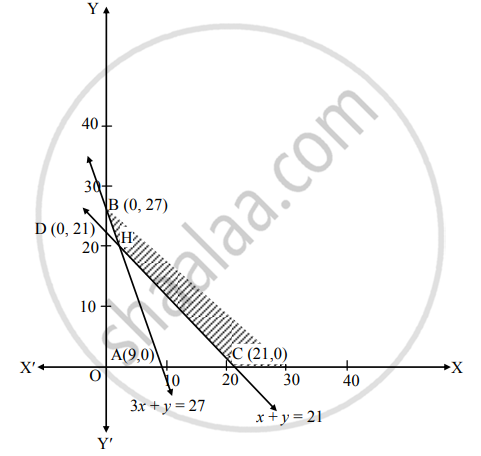# Solve the following problem : Minimize Z = 4x + 2y Subject to 3x + y ≥ 27, x + y ≥ 21, x ≥ 0, y ≥ 0 - Mathematics and Statistics

Graph

Solve the following problem :

Minimize Z = 4x + 2y Subject to 3x + y ≥ 27, x + y ≥ 21, x ≥ 0, y ≥ 0

#### Solution

To find the graphical solution, construct the table as follows:

 Inequation equation Double intercept form Points (x1, x2) Points (x1, x2) 3x + y ≥ 27 3x + y = 27 x/(9) + y/(27) = 1 A (9, 0) B (0, 27) 3(0) + 0 ≥ 27∴ 0 ≥ 27∴ non-origin-side x + y ≥ 21 x + y = 21 x/(21) + y/(21) = 1 C (21, 0) D (0, 21) (0) + 0 ≥ 21∴ 0 ≥ 21∴ non-origin-side x ≥ 0 x = 0 – R.H.S. of Y-axis y ≥ 0 y = 0 above X-axisThe shaded portion CHB is the feasible region.
Whose vertices are C(21, 0), H and B(0, 27)
H is the point of intersection of lines
3x + y = 27 …(i)
x + y = 21  …(ii)
∴ By (i) – (ii), we get
3 x + y = 27
x + y = 21
–    –     –
2x        = 6

∴ x = (6)/(2)  = 3
Substituting x = 3 in (ii), we get
3 + y = 21
∴ y = 18
∴ H (3, 18)
Here, the objective function is Z = 4x + 2y
Now, we will find minimum value of Z as follows:

 Feasible points The value of Z = 4x + 2y C (21, 0) Z = 4(21) + 2(0) = 84 H (3, 18) Z = 4(3) + 2(18) = 12 + 36 = 48 B (0, 27) Z = 4(0) + 2(27) = 54

∴ Z has minimum value 48 at H (3, 18)
∴ Z is minimum, when x = 3, y = 18

Is there an error in this question or solution?
Chapter 6: Linear Programming - Miscellaneous Exercise 6 [Page 104]

#### APPEARS IN

Balbharati Mathematics and Statistics 2 (Commerce) 12th Standard HSC Maharashtra State Board
Chapter 6 Linear Programming
Miscellaneous Exercise 6 | Q 4.02 | Page 104
Share